Скачать презентацию Chapters 14 15 Security The Security Environment

1cbb57cc9657ce78d852509e3994c4cd.ppt

• Количество слайдов: 28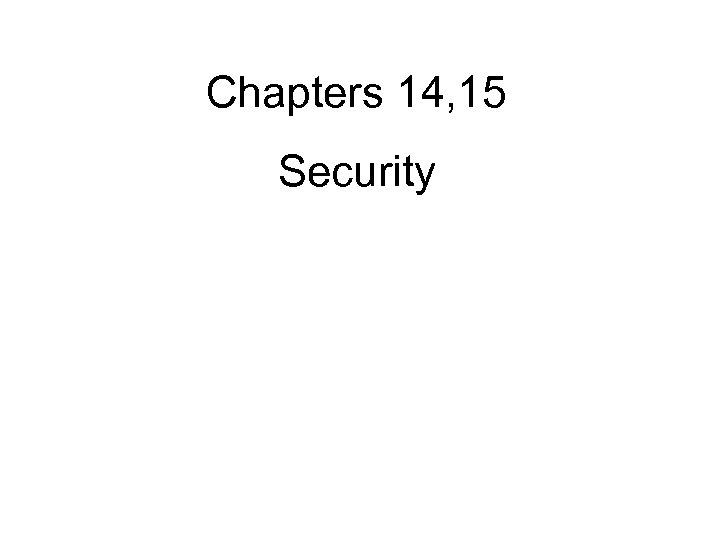Chapters 14, 15 Security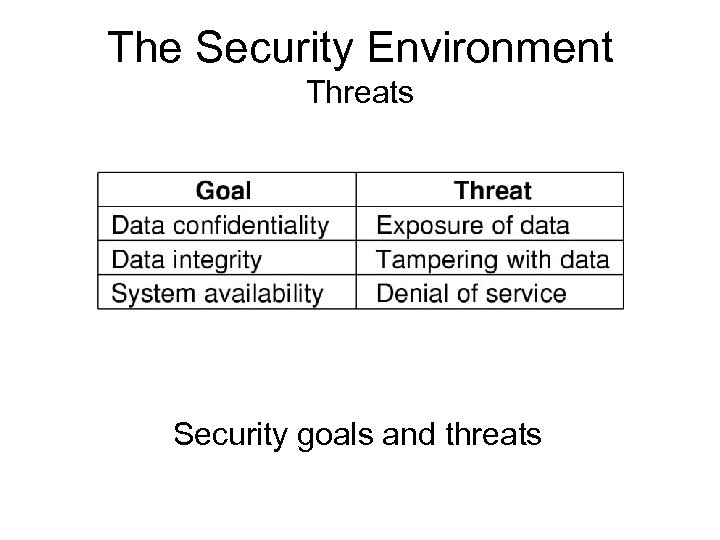The Security Environment Threats Security goals and threats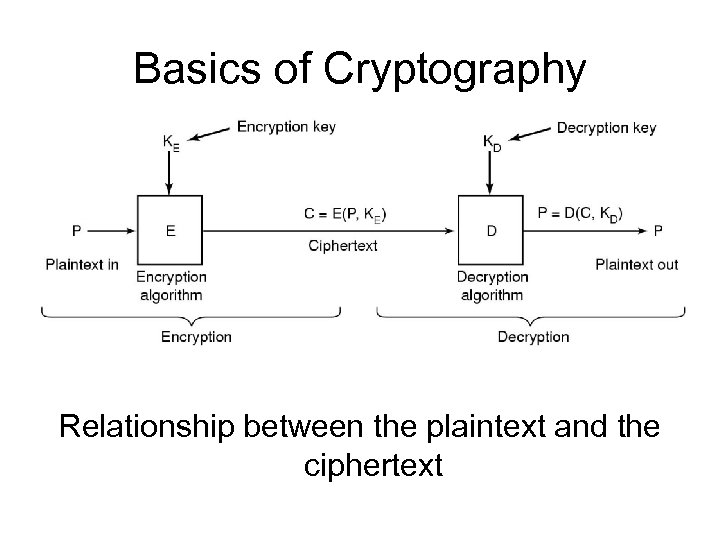Basics of Cryptography Relationship between the plaintext and the ciphertext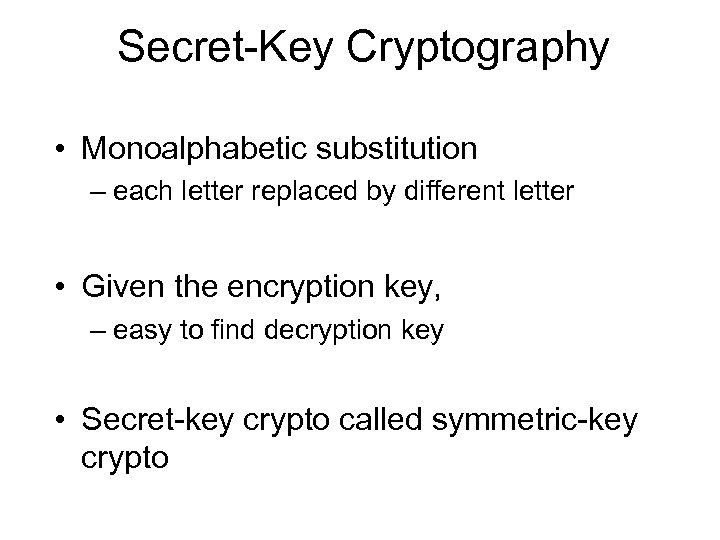Secret-Key Cryptography • Monoalphabetic substitution – each letter replaced by different letter • Given the encryption key, – easy to find decryption key • Secret-key crypto called symmetric-key crypto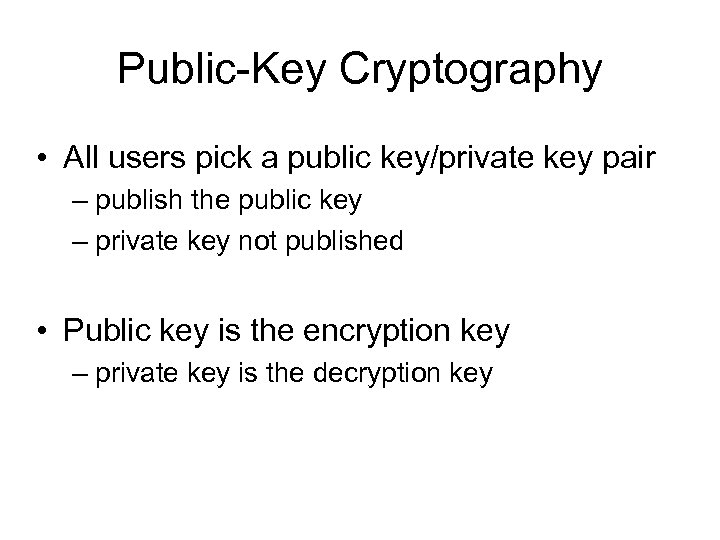Public-Key Cryptography • All users pick a public key/private key pair – publish the public key – private key not published • Public key is the encryption key – private key is the decryption key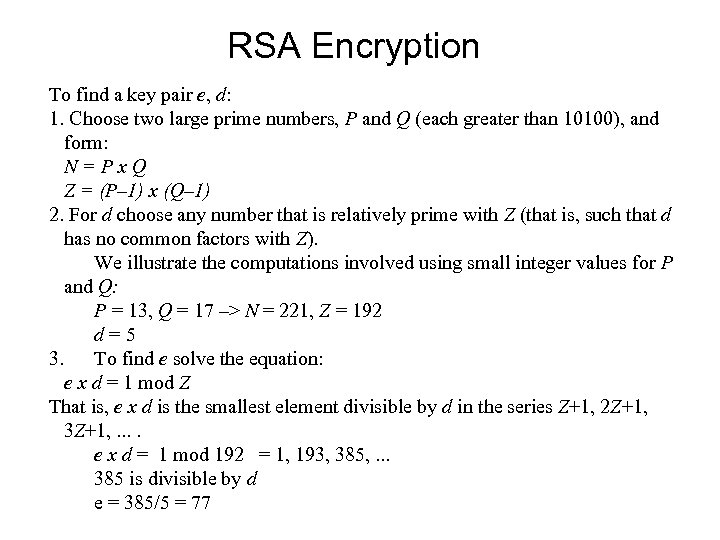RSA Encryption To find a key pair e, d: 1. Choose two large prime numbers, P and Q (each greater than 10100), and form: N=Px. Q Z = (P– 1) x (Q– 1) 2. For d choose any number that is relatively prime with Z (that is, such that d has no common factors with Z). We illustrate the computations involved using small integer values for P and Q: P = 13, Q = 17 –> N = 221, Z = 192 d=5 3. To find e solve the equation: e x d = 1 mod Z That is, e x d is the smallest element divisible by d in the series Z+1, 2 Z+1, 3 Z+1, . . e x d = 1 mod 192 = 1, 193, 385, . . . 385 is divisible by d e = 385/5 = 77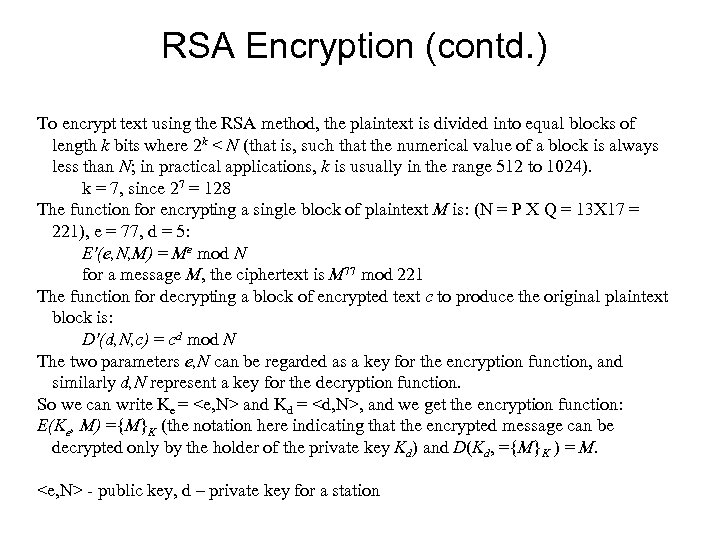RSA Encryption (contd. ) To encrypt text using the RSA method, the plaintext is divided into equal blocks of length k bits where 2 k < N (that is, such that the numerical value of a block is always less than N; in practical applications, k is usually in the range 512 to 1024). k = 7, since 27 = 128 The function for encrypting a single block of plaintext M is: (N = P X Q = 13 X 17 = 221), e = 77, d = 5: E'(e, N, M) = Me mod N for a message M, the ciphertext is M 77 mod 221 The function for decrypting a block of encrypted text c to produce the original plaintext block is: D'(d, N, c) = cd mod N The two parameters e, N can be regarded as a key for the encryption function, and similarly d, N represent a key for the decryption function. So we can write Ke = and Kd = , and we get the encryption function: E(Ke, M) ={M}K (the notation here indicating that the encrypted message can be decrypted only by the holder of the private key Kd) and D(Kd, ={M}K ) = M. - public key, d – private key for a station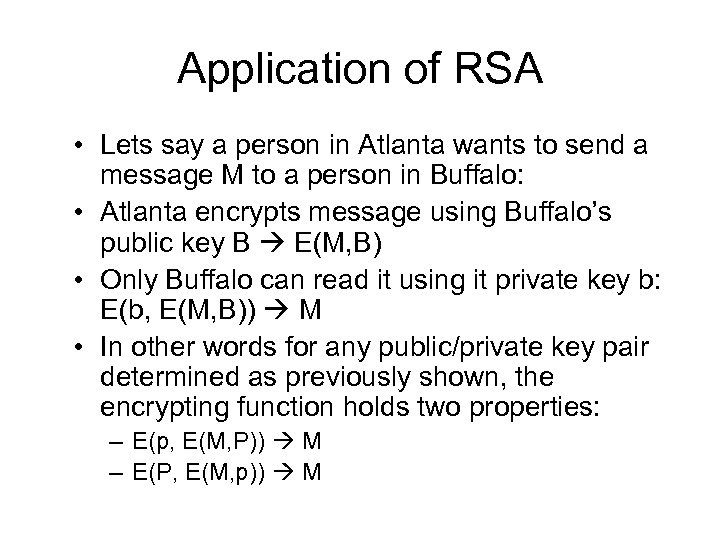Application of RSA • Lets say a person in Atlanta wants to send a message M to a person in Buffalo: • Atlanta encrypts message using Buffalo’s public key B E(M, B) • Only Buffalo can read it using it private key b: E(b, E(M, B)) M • In other words for any public/private key pair determined as previously shown, the encrypting function holds two properties: – E(p, E(M, P)) M – E(P, E(M, p)) M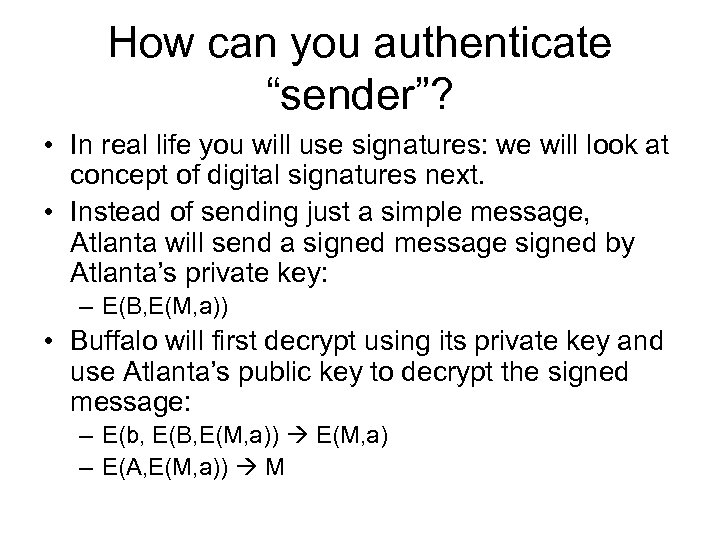How can you authenticate “sender”? • In real life you will use signatures: we will look at concept of digital signatures next. • Instead of sending just a simple message, Atlanta will send a signed message signed by Atlanta’s private key: – E(B, E(M, a)) • Buffalo will first decrypt using its private key and use Atlanta’s public key to decrypt the signed message: – E(b, E(B, E(M, a)) E(M, a) – E(A, E(M, a)) M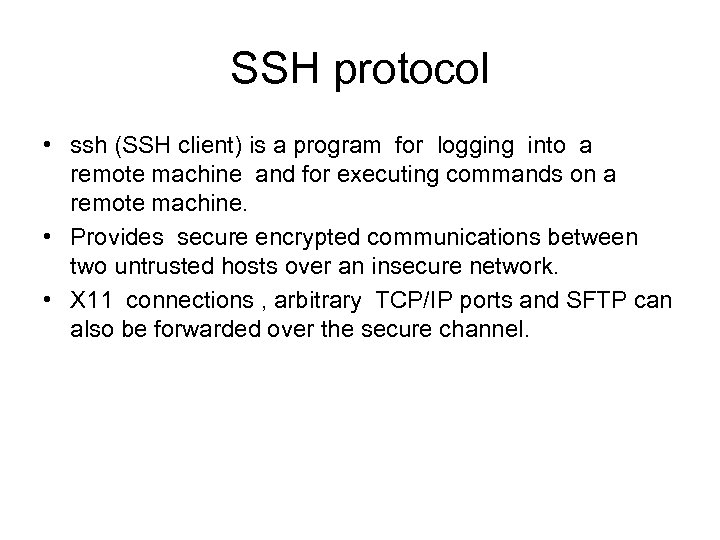SSH protocol • ssh (SSH client) is a program for logging into a remote machine and for executing commands on a remote machine. • Provides secure encrypted communications between two untrusted hosts over an insecure network. • X 11 connections , arbitrary TCP/IP ports and SFTP can also be forwarded over the secure channel.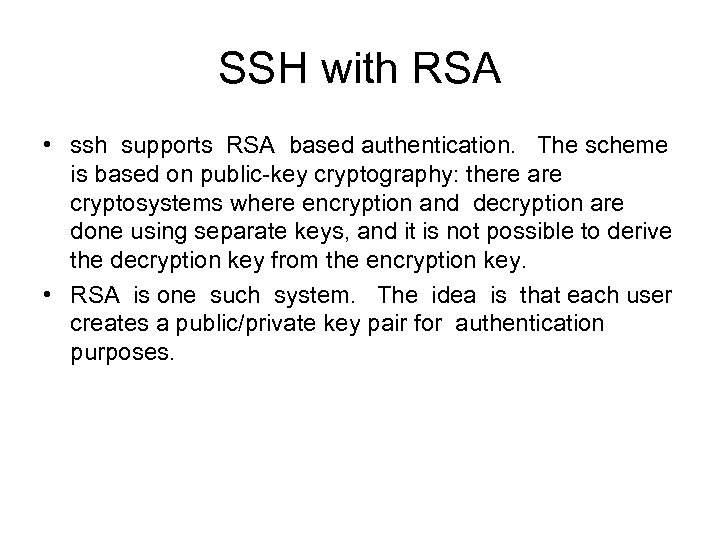SSH with RSA • ssh supports RSA based authentication. The scheme is based on public-key cryptography: there are cryptosystems where encryption and decryption are done using separate keys, and it is not possible to derive the decryption key from the encryption key. • RSA is one such system. The idea is that each user creates a public/private key pair for authentication purposes.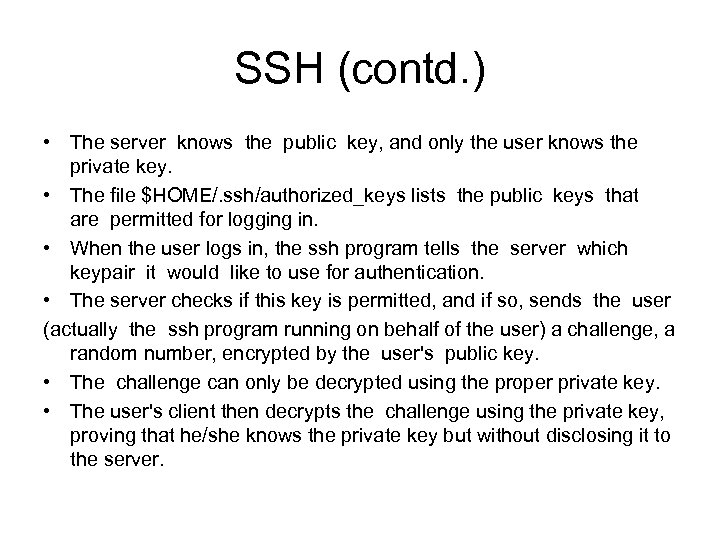SSH (contd. ) • The server knows the public key, and only the user knows the private key. • The file \$HOME/. ssh/authorized_keys lists the public keys that are permitted for logging in. • When the user logs in, the ssh program tells the server which keypair it would like to use for authentication. • The server checks if this key is permitted, and if so, sends the user (actually the ssh program running on behalf of the user) a challenge, a random number, encrypted by the user's public key. • The challenge can only be decrypted using the proper private key. • The user's client then decrypts the challenge using the private key, proving that he/she knows the private key but without disclosing it to the server.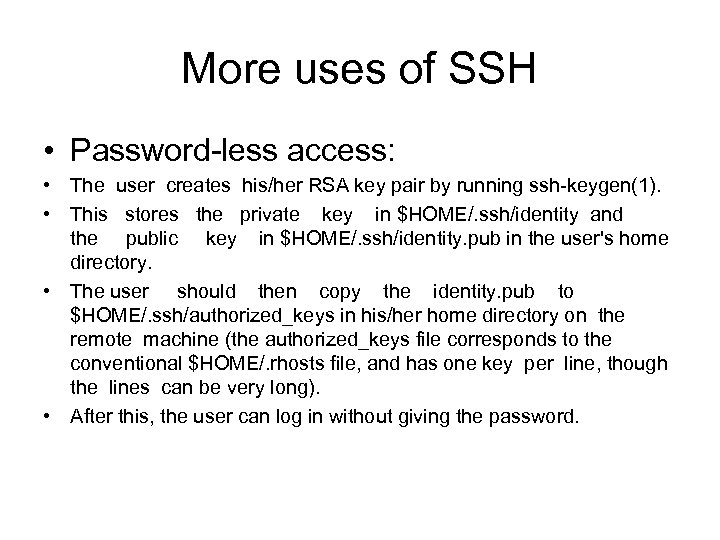More uses of SSH • Password-less access: • The user creates his/her RSA key pair by running ssh-keygen(1). • This stores the private key in \$HOME/. ssh/identity and the public key in \$HOME/. ssh/identity. pub in the user's home directory. • The user should then copy the identity. pub to \$HOME/. ssh/authorized_keys in his/her home directory on the remote machine (the authorized_keys file corresponds to the conventional \$HOME/. rhosts file, and has one key per line, though the lines can be very long). • After this, the user can log in without giving the password.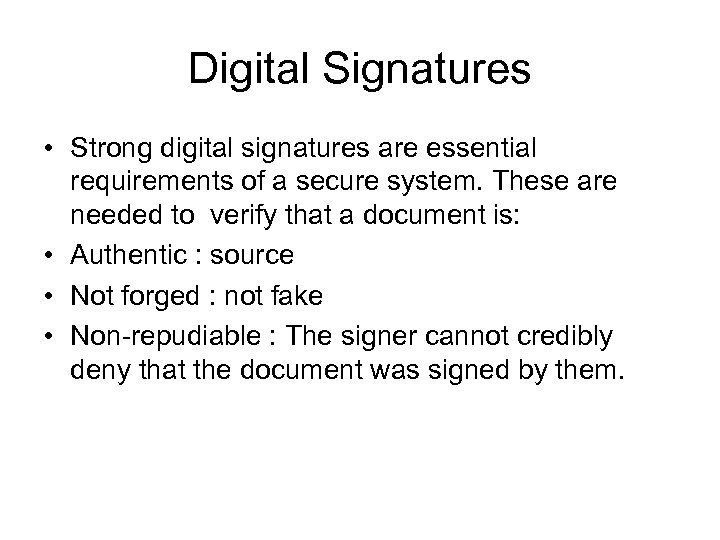Digital Signatures • Strong digital signatures are essential requirements of a secure system. These are needed to verify that a document is: • Authentic : source • Not forged : not fake • Non-repudiable : The signer cannot credibly deny that the document was signed by them.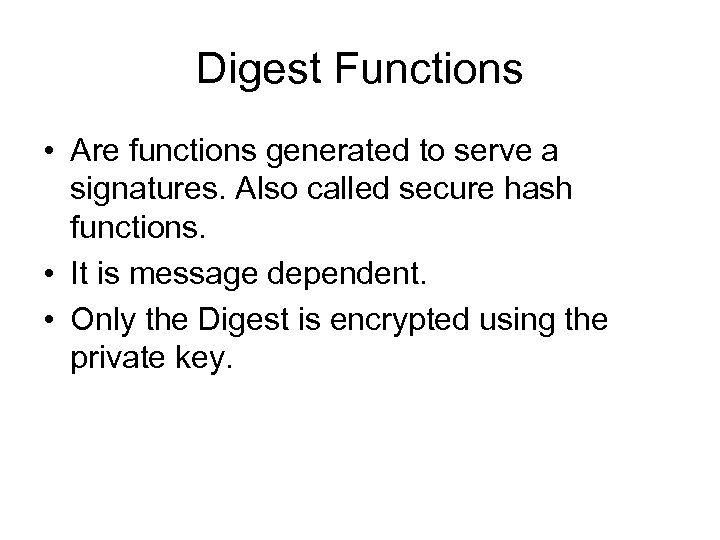Digest Functions • Are functions generated to serve a signatures. Also called secure hash functions. • It is message dependent. • Only the Digest is encrypted using the private key.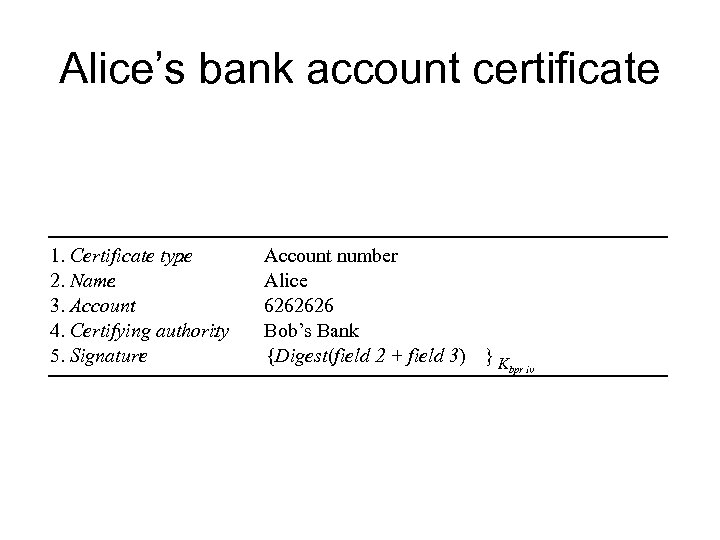Alice’s bank account certificate 1. Certificate type : 2. Name : 3. Account : 4. Certifying authority : 5. Signature : Account number Alice 6262626 Bob’s Bank {Digest(field 2 + field 3) }K bpr iv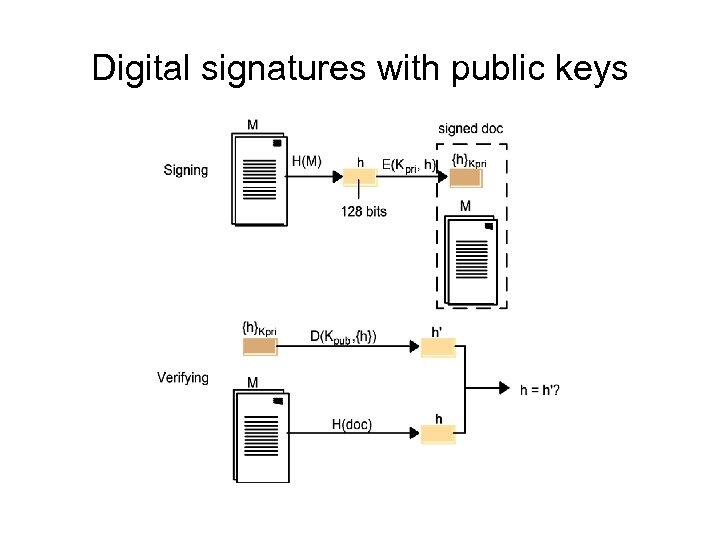Digital signatures with public keys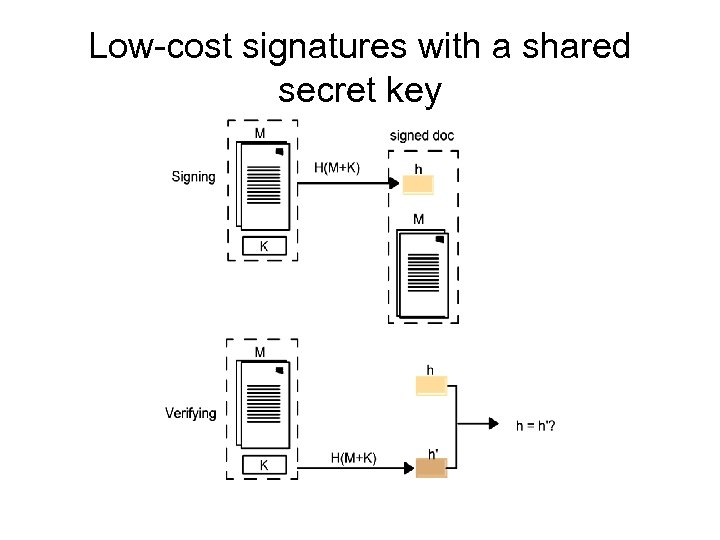Low-cost signatures with a shared secret key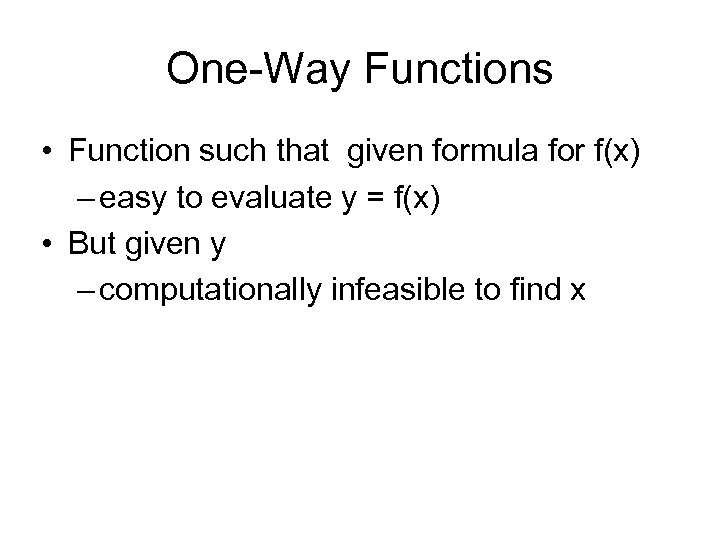One-Way Functions • Function such that given formula for f(x) – easy to evaluate y = f(x) • But given y – computationally infeasible to find x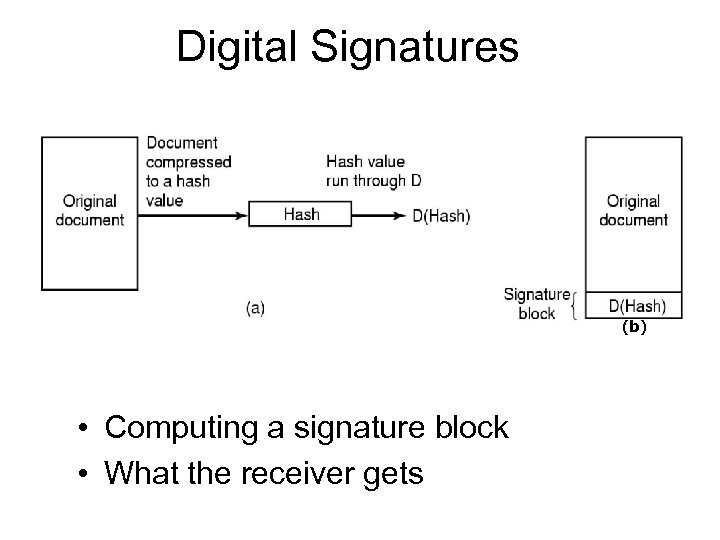Digital Signatures (b) • Computing a signature block • What the receiver gets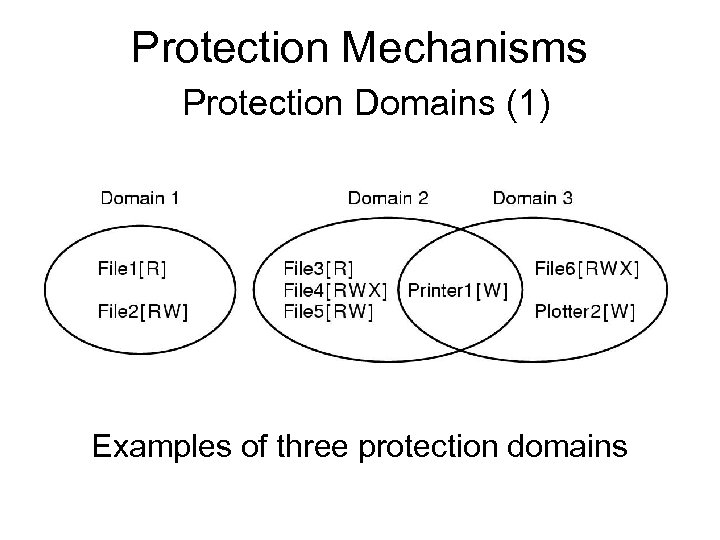Protection Mechanisms Protection Domains (1) Examples of three protection domains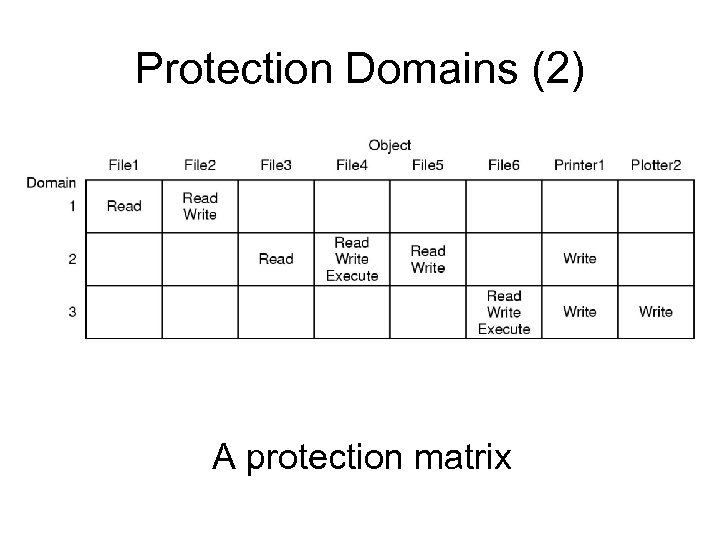Protection Domains (2) A protection matrix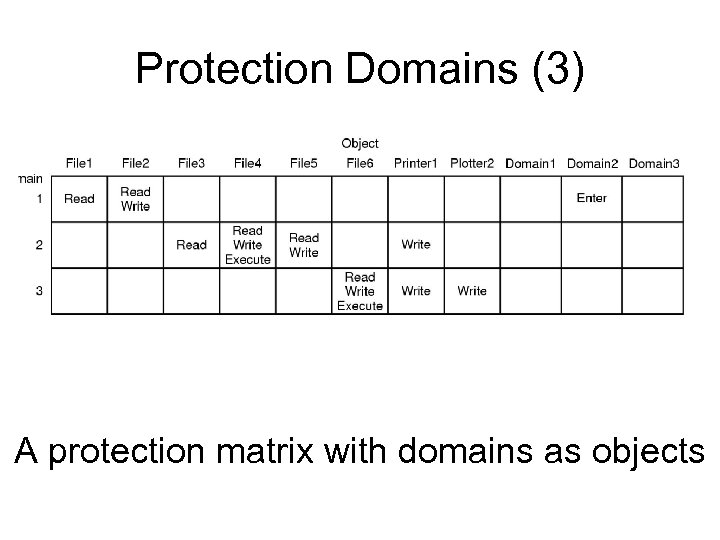Protection Domains (3) A protection matrix with domains as objects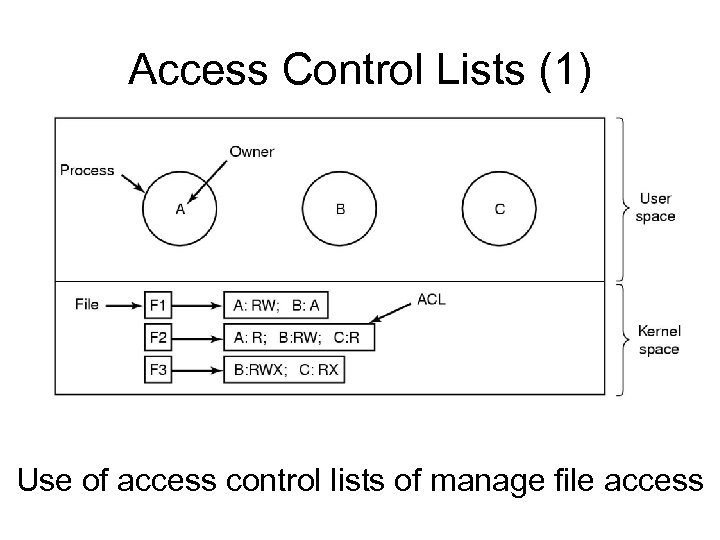Access Control Lists (1) Use of access control lists of manage file access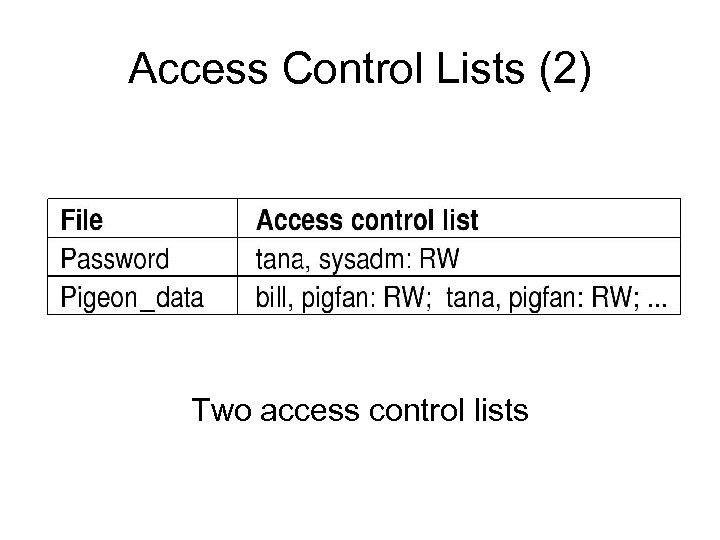Access Control Lists (2) Two access control lists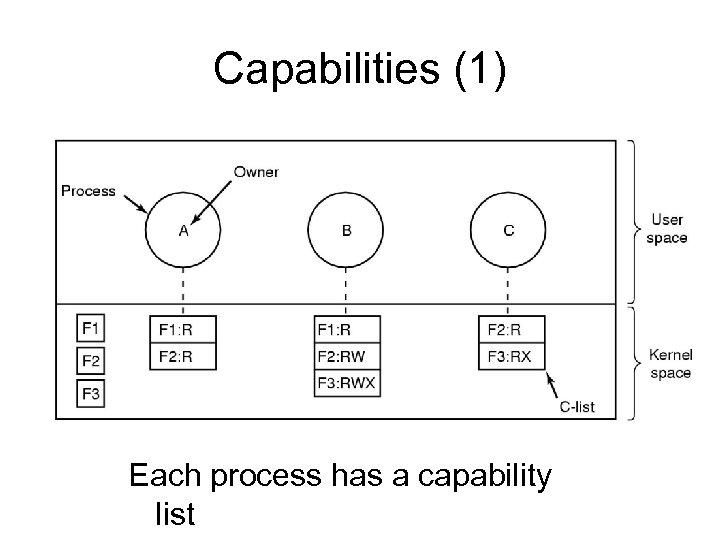Capabilities (1) Each process has a capability list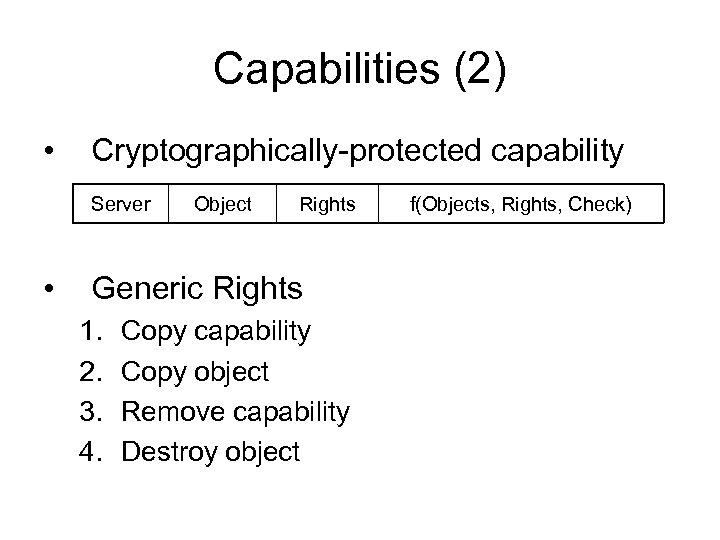Capabilities (2) • Cryptographically-protected capability Server • Object Rights Generic Rights 1. 2. 3. 4. Copy capability Copy object Remove capability Destroy object f(Objects, Rights, Check)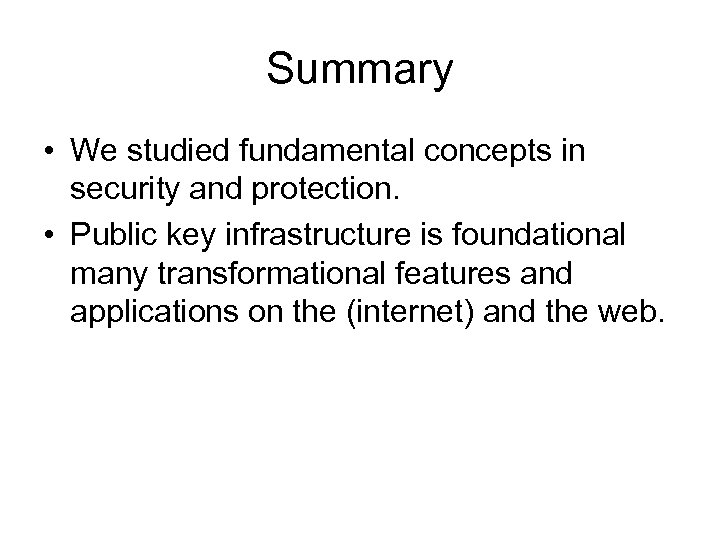Summary • We studied fundamental concepts in security and protection. • Public key infrastructure is foundational many transformational features and applications on the (internet) and the web.